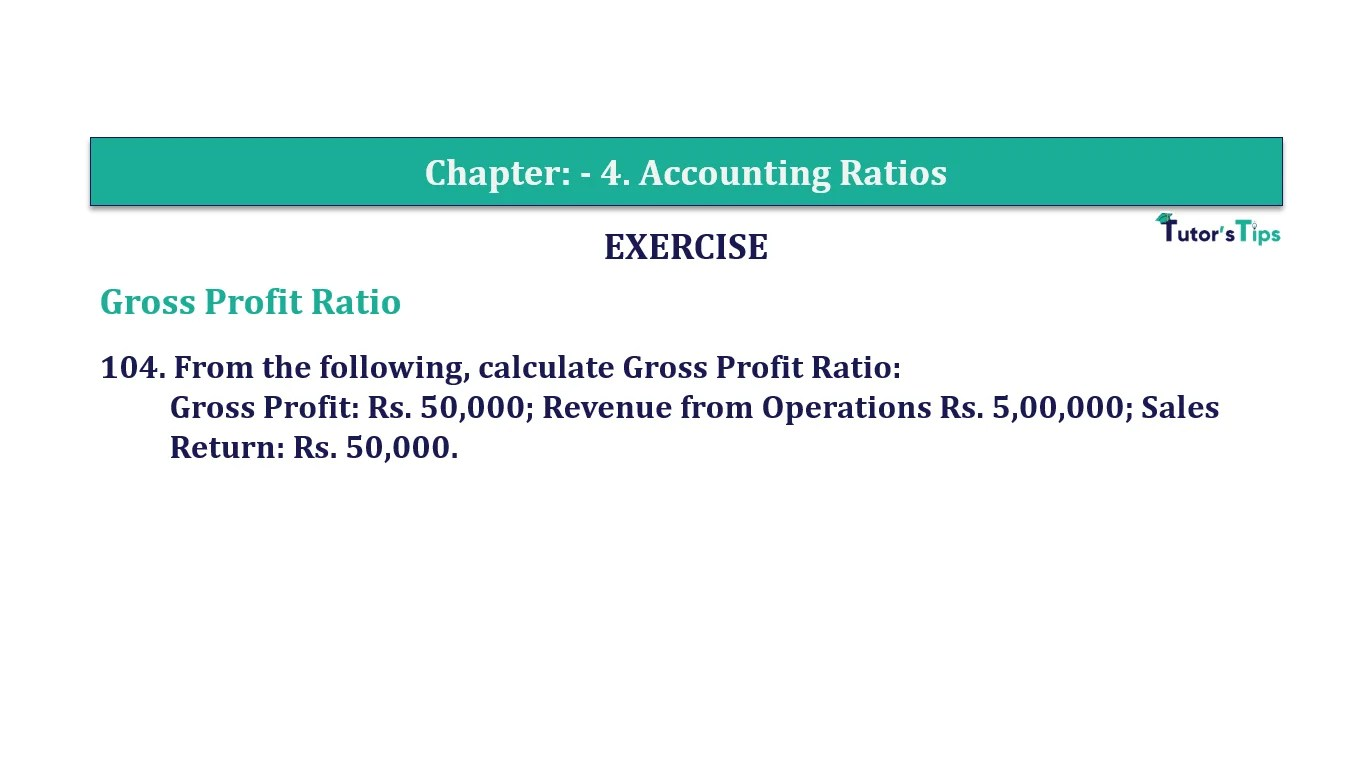# Question 104 Chapter 4 of +2-B – T.S. Grewal 12 ClassQuestion No. 104- Chapter No.4 - T.S. Grewal +2 Book Part B

Question 104 Chapter 4 of +2-B

Gross Profit Ratio

104. From the following, calculate Gross Profit Ratio:
Gross Profit: Rs. 50,000; Revenue from Operations Rs. 5,00,000; Sales
Return: Rs. 50,000.

### The solution of Question 104 Chapter 4 of +2-B: –

 Net Sales = Rs. 5,00,000 Gross Profit = Rs. 50,000

 Revenue from Operations = Gross Profit X 100 Revenue from Operations
 Revenue from Operations = 50,000 X 100 5,00,000 = 10%

Balance Sheet: Meaning, Format & Examples

Comment if you have any question.

Also, Check out the solved question of previous Chapters: –